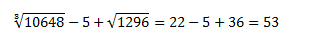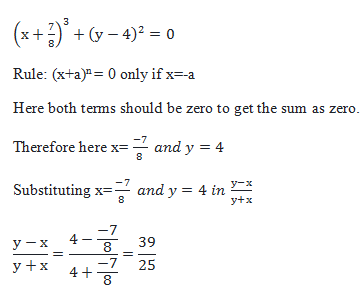# SSC CGL 2018 Practice Test Papers | Quantitative Aptitude (Day-50)

Dear Aspirants, Here we have given the Important SSC CGL Exam 2018 Practice Test Papers. Candidates those who are preparing for SSC CGL 2018 can practice these questions to get more confidence to Crack SSC CGL 2018 Examination.

[WpProQuiz 3539]

Click “Start Quiz” to attend these Questions and view Explanation

1) Ratio of ages of A and B is 2 : 5 and that of A and C is 3:7. If the difference in the ages of A and C is 32 years, find the sum of age of B and C.

a) 120

b) 116

c) 130

d) 143

2)  Age of Asmitha after 20 years will be 2 times of her age before 5 years. What is the present age of Asmitha?

a) 30

b) 25

c) 20

d) 35

3) A can do 75% of a job in 27 days and B can do 25% of the job in 24 days. If they work on it together, in how many days can they do 50% of the job?

a) 144/11

b) 134/11

c) 342/12

d) None of these

4) Aarthi was asked to find the area of four walls of a cuboid. Its length, breadth and height are 10 cm, 7.5 cm and 6 cm respectively. What is the area of four walls of a cuboid?

a) 550 cu.cm

b) 660 cu.cm

c) 420 cu. cm

d) 350 cu.cm

5) What is the average of all positive numbers between 120 and 240 which are divisible by 8?

a) 200

b) 180

c) 250

d) 300

6) A trader had 540 kg of rice. He sold a part of it at 20% profit and the rest at 11% profit, so that he made a total profit of 15%. How much rice (in kg) did he sell at 11% profit?

a) 300 kg

b) 240 kg

c) 440 kg

d) 400 kg

7) If 60% of X = Z, then 30% of Z is equal to ___ of X.

a) 0.2

b) 0.18

c) 0.5

d) 0.7

8) What is the reflection of the point (−4, 7) in the line y = 1?

a) (4, −5)

b) (−4, −5)

c) (3, 7)

d) (−4, 5)

9) Find the value of 10648 – 5 √1296

a) 27

b) 30

c) 53

d) 45

10)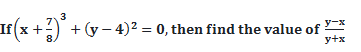a) 39/11

b) 39/25

c) 25/42

d) None of the above

A: B = 2: 5 = 6: 15

A: C = 3:7 = 6:14

A: B: C = 6:15:14

14x – 6x = 8x

8x = 32

X = 4 years

Sum of age of B and C = 15x + 14x = 29x = 29 × 4 = 116

Let the age of Asmitha be x years

Age of Asmitha after 20 years= x+20 years

Age of Asmitha before 5 years= x-5

x + 20 = 2(x – 5)

x + 20 = 2x – 10

x = 30Area of four walls of a cuboid = 2h (l+b)

= 2×6(10+17.5)

= 660 cu. cm

Divisors of 8 between 120 and 240 are

120, 128, 136, …, 240.

This is in A.P. a = 120, d = 8 and Tn = 240.

Tn = a + (n – 1) d

240 = 120 + (n – 1) × 8

=>120 = (n – 1) × 8

N – 1 = 15.

N = 16

Sum of n terms of AP = n/2(2a+ (n – 1) d)

=16/2(2×120 + (16 – 1) 8)

=2880

Average of 16 terms = 2880/16 = 180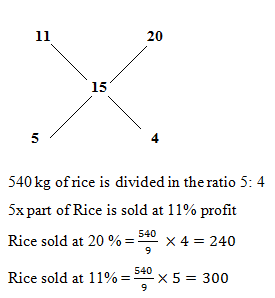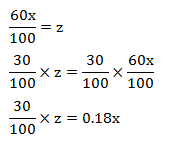Y = 1 is a horizontal line.
The y value is 6 points above the horizontal line (y = 1), so calculate 6 points above the horizontal line. So the reflected point will be (-4, 5).